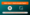# CBSE Class 11 Maths Sample Papers | Download Sample Papers PDFsCBSE Class 11 Maths Sample Papers are one of the best study materials to prepare well for the exam and score good marks. The students can get complete knowledge about whole maths chapters while practicing these sample papers.

Sample Question Papers are created by the experts and as per the CBSE 11th Maths Syllabus guidelines. These solutions are given in detail that covers each chapter so that the students can easily understand the concept.

Here you will get the detailed guide on CBSE Class 11 Maths Sample Papers.

## CBSE Class 11 Maths Sample Papers

11th Maths Sample Question Papers enable the students to understand the exam pattern and also assist them to make their strong foundation in maths subject. These sample papers also assist the students to get confident to face any difficult questions asked in the exam.

The students should practice Question Papers on a daily basis in order to score high marks. They can focus on their weak points with the help of these sample papers. They can also examine how much they are prepared for the exam.

The students can easily and conveniently download the 11th Maths Sample Question Papers to understand the pattern of questions asks in the board exam. The students should practice various maths sample papers regularly for a better understanding of concepts and score good marks.

Here are providing 11th maths sample papers with solutions for academic years as follows:Download Ultimate Study Materials to Boost Your Preparation CBSE Class 11 Maths Study Materials CBSE 11th Maths Books CBSE Guide Class 11th Class 11 goUnlimited Ebooks

Practicing Question Papers allow the students to know the exam pattern and the time to answer every question. The students can effortlessly download CBSE class 11 maths sample paper 2020 PDF for their reference that assists the students to score high ranks in their exams and make a foundation for class 12. Solving Sample Question Papers is a good way to prepare for the final exam as it raises the confidence of the students.

## Benefits of CBSE Class 11 Maths Sample Papers

Notable Benefits of Solving CBSE 11th Maths Sample Papers

1. 11th Maths Sample Question Papers provide students the best practice for the exam to score high marks.
2. 11th Maths Sample Question Papers with solutions assist the students to identify their weakness, remove silly mistakes, and focus more on their strong areas.
3. 11th Maths Sample Question Papers with solutions enables the students get confident for solving the exam paper on time with accurate answers.
4. 11th Maths Sample Question Papers PDF is provided for each chapter that helps students to self evaluate their answers as well.
5. The students can get a clear idea about the exam paper pattern.
6. They can easily access the 11th Maths Sample Question Papers PDF
7. 11th Maths Sample Question Papers with solutions available in PDF format and in online as well offline mode.
8. These sample papers help the students to understand the marking scheme and good for self-evaluation.
9. 11th Maths Sample Question Papers are prepared according to the latest CBSE syllabus pattern and guidelines.

We have covered the detailed guide on CBSE Class 11 Maths Sample Papers. Feel free to ask any questions in the comment section below.